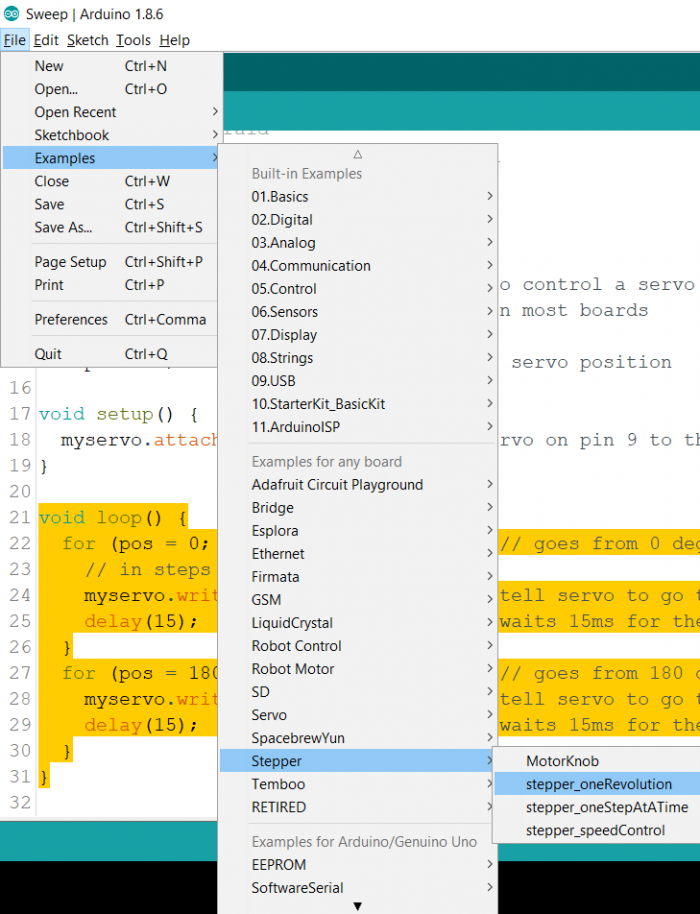# Controlling a Stepper Motor with Arduino

ArduinoArduino BoardsArduino IDEArduino Programming Language

A stepper motor divides the full rotation into a number of discrete steps, ranging from as low as 12 to as high as 200 steps per revolution (corresponding to angles of 30 degrees per step to 1.8 degrees per step). While a DC motor rotates continuously, a stepper motor rotates discretely, in step angles.

## Circuit Diagram

The circuit diagram and the required components for both Unipolar and Bipolar stepper motors can be found here − https://www.arduino.cc/en/Tutorial/LibraryExamples/StepperOneRevolution

Note that the stepper motor is connected to pins 8-11 of Arduino Uno, via a Darlington Array (for unipolar stepper) or H-bridge (for bipolar stepper). The stepper motor is powered using an external supply as it draws too much power to be directly powered from the Arduino board.

## Code Walkthrough

We will be walking through an example code. Go to File → Examples → Stepper → stepper_oneRevolution.Alternatively, the code can be found on GitHub − https://github.com/arduinolibraries/Stepper/blob/master/examples/stepper_oneRevolution/stepper_oneRevolution.ino

Through this code, we make the stepper motor rotate one revolution in one direction, and another revolution in the opposite direction.

We begin with the inclusion of the Stepper library and defining the number of steps per revolution (change this according to the specification of your stepper motor)

#include <Stepper.h>

const int stepsPerRevolution = 200;

Next, we initialize the Stepper object using the stepsPerRevolution and the four pins of the Arduino which are connected to the Stepper motor.

Stepper myStepper(stepsPerRevolution, 8, 9, 10, 11);

Within the setup, we set the speed of the stepper motor in RPM, and initialize Serial.

void setup() {
// set the speed at 60 rpm:
myStepper.setSpeed(60);
// initialize the serial port:
Serial.begin(9600);
}

Within the loop, we make the motor step stepsPerRevolution in one direction, and then step stepsPerRevolution in the opposite direction (by just appending a negative sign)

void loop() {
// step one revolution in one direction:
Serial.println("clockwise");
myStepper.step(stepsPerRevolution);
delay(500);

// step one revolution in the other direction:
Serial.println("counterclockwise");
myStepper.step(-stepsPerRevolution);
delay(500);
}